User’s Guide : Estimation and Solution Options : Optimization Algorithms

Optimization Algorithms
Given the importance of the proper setting of EViews estimation options, it may prove useful to review briefly various basic optimization algorithms used in nonlinear estimation. Recall that the problem faced in non-linear estimation is to find the values of parameters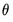that optimize (maximize or minimize) an objective function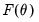.
Iterative optimization algorithms work by taking an initial set of values for the parameters, say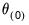, then performing calculations based on these values to obtain a better set of parameter values,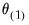. This process is repeated for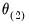,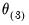and so on until the objective function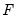no longer improves between iterations.
There are three main parts to the optimization process: (1) obtaining the initial parameter values, (2) updating the candidate parameter vectorat each iteration, and (3) determining when we have reached the optimum.
If the objective function is globally concave so that there is a single maximum, any algorithm which improves the parameter vector at each iteration will eventually find this maximum (assuming that the size of the steps taken does not become negligible). If the objective function is not globally concave, different algorithms may find different local maxima, but all iterative algorithms will suffer from the same problem of being unable to tell apart a local and a global maximum.
The main thing that distinguishes different algorithms is how quickly they find the maximum. Unfortunately, there are no hard and fast rules. For some problems, one method may be faster, for other problems it may not. EViews provides different algorithms, and will often let you choose which method you would like to use.
The following sections outline these methods. The algorithms used in EViews may be broadly classified into three types: second derivative methods, first derivative methods, and derivative free methods. EViews’ second derivative methods evaluate current parameter values and the first and second derivatives of the objective function for every observation. First derivative methods use only the first derivatives of the objective function during the iteration process. As the name suggests, derivative free methods do not compute derivatives.
Second Derivative Methods
For binary, ordered, censored, and count models, EViews can estimate the model using Newton-Raphson or quadratic hill-climbing.
Newton-Raphson
Candidate values for the parametersmay be obtained using the method of Newton-Raphson by linearizing the first order conditions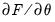at the current parameter values,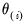: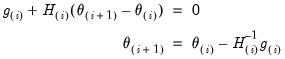(59.14)
where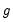is the gradient vector, and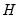is the Hessian matrix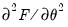.
If the function is quadratic, Newton-Raphson will find the maximum in a single iteration. If the function is not quadratic, the success of the algorithm will depend on how well a local quadratic approximation captures the shape of the function.
This method, which is a straightforward variation on Newton-Raphson, is sometimes attributed to Goldfeld and Quandt. Quadratic hill-climbing modifies the Newton-Raphson algorithm by adding a correction matrix (or ridge factor) to the Hessian. The quadratic hill-climbing updating algorithm is given by: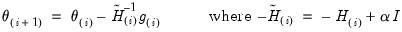(59.15)
where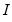is the identity matrix and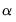is a positive number that is chosen by the algorithm.
The effect of this modification is to push the parameter estimates in the direction of the gradient vector. The idea is that when we are far from the maximum, the local quadratic approximation to the function may be a poor guide to its overall shape, so we may be better off simply following the gradient. The correction may provide better performance at locations far from the optimum, and allows for computation of the direction vector in cases where the Hessian is near singular.
For models which may be estimated using second derivative methods, EViews uses quadratic hill-climbing as its default method. You may elect to use traditional Newton-Raphson, or the first derivative methods described below, by selecting the desired algorithm in the Options menu.
Note that asymptotic standard errors are always computed from the unmodified Hessian once convergence is achieved.
First Derivative Methods
Second derivative methods may be computationally costly since we need to evaluate the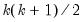elements of the second derivative matrix at every iteration. Moreover, second derivatives calculated may be difficult to compute accurately. An alternative is to employ methods which require only the first derivatives of the objective function at the parameter values.
For selected other nonlinear models (ARCH and GARCH, GMM, State Space), EViews provides two first derivative methods: Gauss-Newton/BHHH or Marquardt.
Nonlinear single equation and system models are estimated using the Marquardt method.
Gauss-Newton/BHHH
This algorithm follows Newton-Raphson, but replaces the negative of the Hessian by an approximation formed from the sum of the outer product of the gradient vectors for each observation’s contribution to the objective function. For least squares and log likelihood functions, this approximation is asymptotically equivalent to the actual Hessian when evaluated at the parameter values which maximize the function. When evaluated away from the maximum, this approximation may be quite poor.
The algorithm is referred to as Gauss-Newton for general nonlinear least squares problems, and often attributed to Berndt, Hall, Hall and Hausman (BHHH) for maximum likelihood problems.
The advantages of approximating the negative Hessian by the outer product of the gradient are that (1) we need to evaluate only the first derivatives, and (2) the outer product is necessarily positive semi-definite. The disadvantage is that, away from the maximum, this approximation may provide a poor guide to the overall shape of the function, so that more iterations may be needed for convergence.
Marquardt
The Marquardt algorithm modifies the Gauss-Newton algorithm in exactly the same manner as quadratic hill climbing modifies the Newton-Raphson method (by adding a correction matrix (or ridge factor) to the Hessian approximation).
The ridge correction handles numerical problems when the outer product is near singular and may improve the convergence rate. As above, the algorithm pushes the updated parameter values in the direction of the gradient.
For models which may be estimated using first derivative methods, EViews uses Marquardt as its default method. In many cases, you may elect to use traditional Gauss-Newton via the Options menu.
Note that asymptotic standard errors are always computed from the unmodified (Gauss-Newton) Hessian approximation once convergence is achieved.
Choosing the step size
At each iteration, we can search along the given direction for the optimal step size. EViews performs a simple trial-and-error search at each iteration to determine a step size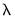that improves the objective function. This procedure is sometimes referred to as squeezing or stretching.
Note that while EViews will make a crude attempt to find a good step,is not actually optimized at each iteration since the computation of the direction vector is often more important than the choice of the step size. It is possible, however, that EViews will be unable to find a step size that improves the objective function. In this case, EViews will issue an error message.
EViews also performs a crude trial-and-error search to determine the scale factorfor Marquardt and quadratic hill-climbing methods.
Derivative free methods
Other optimization routines do not require the computation of derivatives. The grid search is a leading example. Grid search simply computes the objective function on a grid of parameter values and chooses the parameters with the highest values. Grid search is computationally costly, especially for multi-parameter models.
EViews uses (a version of) grid search for the exponential smoothing routine.